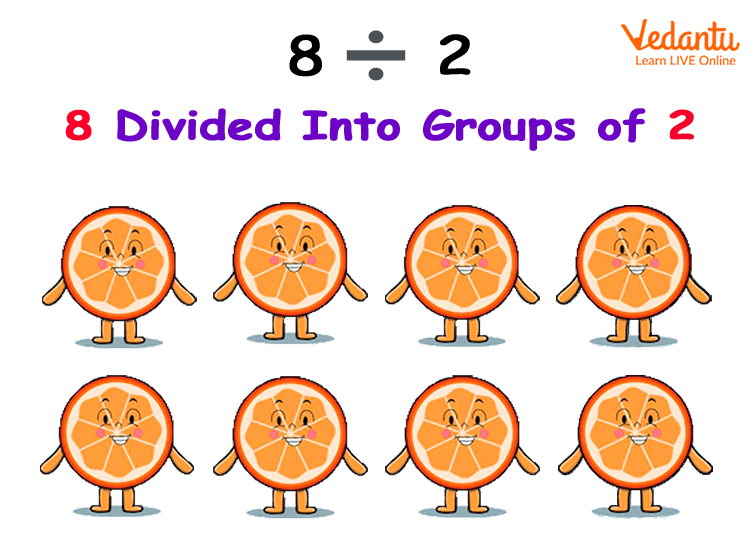Courses
Courses for Kids
Free study material
Free LIVE classes
More

# The Concept of Quotative Division in MathLIVE
Join Vedantu’s FREE Mastercalss

The quotative division in math is defined as dividing the number into groups.

Let us assume that a student has 48 toffees that he has to distribute among his classmates. One day, he noticed that some students were absent and he had to distribute two extra toffees to each, so how many students were there in original?

Well, for dividing the toffees among his classmates, he needs to know the concept of division? So, how will I be doing this? Quite complicated right? Well, let us take a simple example.

Let us say he has 8 toffees and he has to distribute them between two of his friends, so each friend gets four toffees, now this is the simple step. While doing a variety of questions we will look at this quotative division concept more clearly.

Now, let us understand what quotative division is with certain examples.

## Examples of Quotative Division

From the above example of toffee distribution, we will understand the division method.How Quotative Division Works?

Example 1: Here, assume that the number of students was 8 and toffees distributed will be 48/8 = 6, so 6 toffees were given to each classmate.

Originally, let us say the number of students was 12, so each student got 48/12 = 4 toffees each.

Here, we notice that originally each student was expected to get 4 toffees, however, 4 students were absent, so each student received 2 toffees extra.

Therefore, the original number of students was 12.

Example 2: Now, let us say that Namya walked 10 km distance from city A to city B, by taking ‘n’ number of equal steps.

Here, the equal number of steps means for 10 km distance, there should be an equal number of kilometer distance so as to reach her destination.

Here, we divide 10/1, we get 10 km again. Now, we divide 10/2, we get 5. Here, 5 can be considered because 5 and 5 are equal, on adding, they give 10 km again.

Now, let us say she takes more than two steps of equal distances to reach 10 km distance. So, let us move ahead:

10/3 doesn’t give equal steps. Now, 10/4 also doesn’t give. Moving forward, we have 10/5 = 2 km. So each step is of say 2 km, so 5 steps of 2 km were taken by Namya to reach 10 km distance.

Hence, our analysis reach the conclusion that Namya took 2 km steps 5 times to cover the distance of 10 km.

From the above text, we understand that when we divide a number into groups of a measured quantity, it is quotative division. From the above examples, we understood how quotative division can be used in real-life as well.

Last updated date: 24th Sep 2023
Total views: 138.6k
Views today: 4.38k

## FAQs on The Concept of Quotative Division in Math

1. Does quotient mean to divide?

The word quotient comes from a Latin word meaning "how many times."

For example, when you divide any two numbers the answer we obtain is the quotient. The quotient of 12 divided by 2 is 6.

2. State helpful definitions used in division.

Below are the definitions useful in the division:

Dividend – A number that we divide by another number.

Divisor – A number that divides the given number.

Quotient – The result that we obtain by dividing one number (dividend) by another number (divisor).

Reminder – A part that is left after dividing the number. If the remainder is zero, the number is completely divisible, otherwise not.